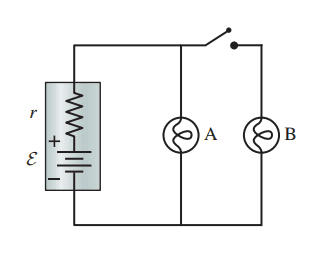# Circuits and the percent change of current when the switch is closed

• ChrisWM

#### ChrisWM

Homework Statement
By what percent does the current through A change when the switch is closed
Relevant Equations
final-initial/initial
For an ideal battery (r = 0 Ω), closing the switch in (Figure 1)does not affect the brightness of bulb A. In practice, bulb A dims just a little when the switch closes. To see why, assume that the 1.50 V battery has an internal resistance r = 0.30 Ω and that the resistance of a glowing bulb is R = 8.00 Ω.Part A: What is the current through bulb A when the switch is open? This answer was .181 A

Part B: What is the current through bulb A after the switch has closed? This answer was .174 A

Part C: By what percent does the current through A change when the switch is closed? This is where I am stuck, I've tried -3.86,-3.87,3.86,3.87,4.02, and -4.02 and they are all wrong. I've done . (174-.181)/.181, and (.181-.174)/.174 and got the answers in the previous sentence, and I tried both positive and negative.